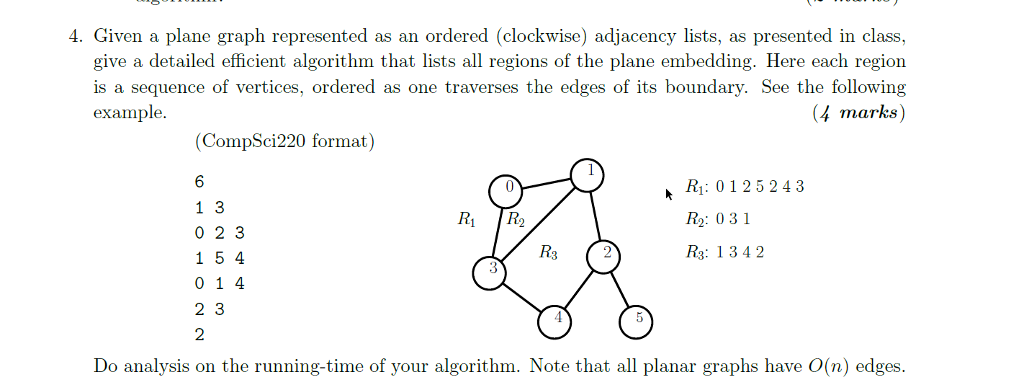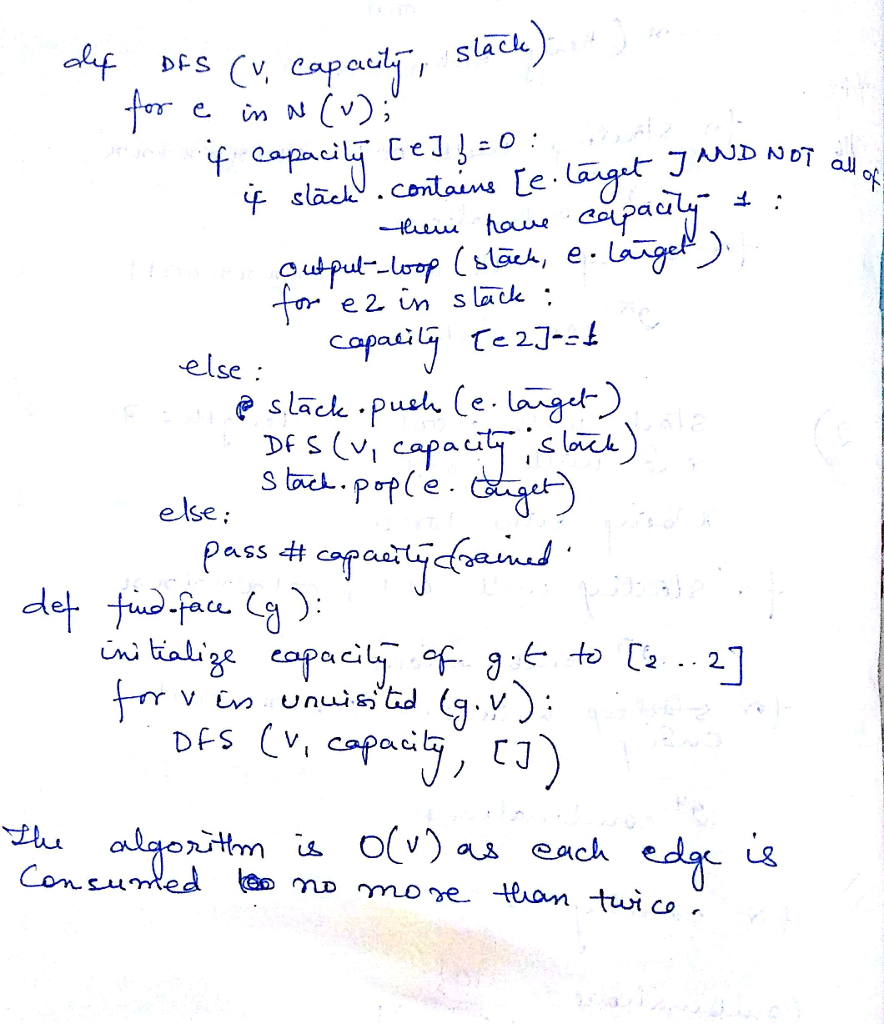# Question & Answer: Given a plane graph represented as an ordered (clockwise) adjacency lists, as presented in class, give a detailed effi…..Given a plane graph represented as an ordered (clockwise) adjacency lists, as presented in class, give a detailed efficient algorithm that lists all regions of the plane embedding. Here each region is a sequence of vertices, ordered as one traverses the edges of its boundary. See the following example. Do analysis on the running-time of your algorithm. Note that all planar graphs have O(n) edges.